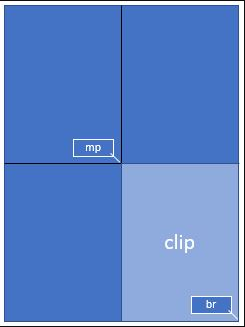### Python将PDF转成图片PNG和JPG——PyMuPDF和pdf2image

2019-08-13  软测小生

1、PDF转PNG图片
2、对PNG图片进行指定区域截图，在另存到指定文件夹下

1、PyMuPDF将PDF转换成图片
pip install PyMuPDF
```
import sys, fitz
import os
import datetime

def pyMuPDF_fitz(pdfPath, imagePath):
startTime_pdf2img = datetime.datetime.now()#开始时间

print("imagePath="+imagePath)
pdfDoc = fitz.open(pdfPath)
for pg in range(pdfDoc.pageCount):
page = pdfDoc[pg]
rotate = int(0)
# 每个尺寸的缩放系数为1.3，这将为我们生成分辨率提高2.6的图像。
# 此处若是不做设置，默认图片大小为：792X612, dpi=96
zoom_x = 1.33333333 #(1.33333333-->1056x816)   (2-->1584x1224)
zoom_y = 1.33333333
mat = fitz.Matrix(zoom_x, zoom_y).preRotate(rotate)
pix = page.getPixmap(matrix=mat, alpha=False)

if not os.path.exists(imagePath):#判断存放图片的文件夹是否存在
os.makedirs(imagePath) # 若图片文件夹不存在就创建

pix.writePNG(imagePath+'/'+'images_%s.png' % pg)#将图片写入指定的文件夹内

endTime_pdf2img = datetime.datetime.now()#结束时间
print('pdf2img时间=',(endTime_pdf2img - startTime_pdf2img).seconds)

if __name__ == "__main__":
pdfPath = '../path/demo.pdf'
imagePath = '../path/image'
pyMuPDF_fitz(pdfPath, imagePath)
```
PDF文档页数超过100页的话需要十几秒，因为先转换成一整张1056X816的图片，再对本地文件中的所有图片进行遍历截图，时间上比较慢,通过查看文档发现：```#下面的这段代码就是想要从一页PDF的中心点为起点截取到右下角的区域，截取整张图的1/4.
>>> mat = fitz.Matrix(2, 2)                  # 在每个方向缩放因子2
>>> rect = page.rect                         # 页面的矩形
>>> mp = rect.tl + (rect.br - rect.tl) * 0.5 # 矩形的中心
>>> clip = fitz.Rect(mp, rect.br)            # 我们想要的剪切区域
>>> pix = page.getPixmap(matrix = mat, clip = clip)```

```import sys, fitz
import os
import datetime

def pyMuPDF_fitz(pdfPath, imagePath):
startTime_pdf2img = datetime.datetime.now()#开始时间

pdfDoc = fitz.open(pdfPath)
for pg in range(pdfDoc.pageCount):
page = pdfDoc[pg]
rotate = int(0)
# 每个尺寸的缩放系数为1.3，这将为我们生成分辨率提高2.6的图像。
# 此处若是不做设置，默认图片大小为：792X612, dpi=96
zoom_x = 1.33333333 #(1.33333333-->1056x816)   (2-->1584x1224)
zoom_y = 1.33333333
mat = fitz.Matrix(zoom_x, zoom_y).preRotate(rotate)
pix = page.getPixmap(matrix=mat, alpha=False)

if not os.path.exists(imagePath):#判断存放图片的文件夹是否存在
os.makedirs(imagePath) # 若图片文件夹不存在就创建

pix.writePNG(imagePath+'/'+'images_%s.png' % pg)#将图片写入指定的文件夹内

endTime_pdf2img = datetime.datetime.now()#结束时间
print('pdf2img时间=',(endTime_pdf2img - startTime_pdf2img).seconds)

def pyMuPDF2_fitz(pdfPath, imagePath):
pdfDoc = fitz.open(pdfPath) # open document
for pg in range(pdfDoc.pageCount): # iterate through the pages
page = pdfDoc[pg]
rotate = int(0)
# 每个尺寸的缩放系数为1.3，这将为我们生成分辨率提高2.6的图像
# 此处若是不做设置，默认图片大小为：792X612, dpi=96
zoom_x = 1.33333333 #(1.33333333-->1056x816)   (2-->1584x1224)
zoom_y = 1.33333333
mat = fitz.Matrix(zoom_x, zoom_y).preRotate(rotate) # 缩放系数1.3在每个维度  .preRotate(rotate)是执行一个旋转
rect = page.rect                         # 页面大小
mp = rect.tl + (rect.bl - (0,75/zoom_x)) # 矩形区域    56=75/1.3333
clip = fitz.Rect(mp, rect.br)            # 想要截取的区域
pix = page.getPixmap(matrix=mat, alpha=False, clip=clip) # 将页面转换为图像
if not os.path.exists(imagePath):
os.makedirs(imagePath)
pix.writePNG(imagePath+'/'+'psReport_%s.png' % pg)# store image as a PNG

if __name__ == "__main__":
pdfPath = '../path/demo.pdf'
imagePath = '../path/image'
#pyMuPDF_fitz(pdfPath, imagePath)#只是转换图片
pyMuPDF2_fitz(pdfPath, imagePath)#指定想要的区域转换成图片```

2、pdf2image 将PDF转换成图片
pdf2image也是个包装器，真正的转换工具是poppler
GitHub地址：https://github.com/Belval/pdf2image ，上面也有相关的配置说明。

1、安装pdf2image: pip install pdf2image
2、Windows安装配置poppler（这里只介绍Windows，Mac和Linux去上面Github地址里面参考官网）
Windows用户必须为Windows安装poppler (http://blog.alivate.com.au/poppler-windows/)，然后将bin/文件夹添加到PATH （开始>输入env>编辑系统环境变量>环境变量...>系统变量>Path）

ERROE:FileNotFoundError: [WinError 2] The system cannot find the file specified
During handling of the above exception, another exception occurred:3、pip install pillow (如果你还没有安装过的话)
```from pdf2image import convert_from_path,convert_from_bytes
import tempfile
from pdf2image.exceptions import (
PDFInfoNotInstalledError,
PDFPageCountError,
PDFSyntaxError
)

def pdf2image2(pdfPath, imagePath, pageNum):
#方法一：
#这会将a.pdf转换成在output文件夹下形如ok_线程id-页码.jpg的一些文件。

#下面的写法直接写入到内存,默认是C:\Users\pppp\AppData\Local\Temp\生成的uuid4名字
images = convert_from_path(pdfPath, dpi=96)
for image in images:
if not os.path.exists(imagePath):
os.makedirs(imagePath)
image.save(imagePath+'/'+'psReport_%s.png' % images.index(image), 'PNG')

#方法二：
for image in images:
if not os.path.exists(imagePath):
os.makedirs(imagePath)
image.save(imagePath+'/'+'psReport_%s.png' % images.index(image), 'PNG')

#方法三，也是最推荐的方法
with tempfile.TemporaryDirectory() as path:
images_from_path = convert_from_path(pdfPath, output_folder=path, dpi=96)
for image in images_from_path:
if not os.path.exists(imagePath):
os.makedirs(imagePath)
image.save(imagePath+'/'+'psReport_%s.png' % images_from_path.index(image), 'PNG')
print(images_from_path)
```

convert_from_path(pdf_path, dpi=200, output_folder=None, first_page=None, last_page=None, fmt='ppm', thread_count=1, userpw=None, use_cropbox=False, strict=False, transparent=False, single_file=False, output_file=str(uuid.uuid4()), poppler_path=None)

convert_from_bytes(pdf_file, dpi=200, output_folder=None, first_page=None, last_page=None, fmt='ppm', thread_count=1, userpw=None, use_cropbox=False, strict=False, transparent=False, single_file=False, output_file=str(uuid.uuid4()), poppler_path=None)

pdf_path --> 要转换的PDF文档路径
dpi -->  DPI中的图像质量（默认为200），Windows默认为96dpi
output_folder --> 将生成的图像写入文件夹（而不是直接写入内存）若是path不做指定的话，path的默认地址是：C:\Users\pppp\AppData\Local\Temp\生成的uuid4。
first_page --> 从哪一页开始转换，默认是PDF的第一页
last_page --> 转换到哪一页，默认是PDF的最后一页
fmt --> 输出图像格式默认格式是ppm，还可以设置为png和jpeg等
userpw -->  PDF的密码（若有密码的话需要添加）
use_cropbox --> 使用cropbox而不是mediabox
strict --> 参数允许您使用自定义类型PDFSyntaxError捕获pdftoppm语法错误
transparent --> 参数允许生成没有背景的图像，而不是通常的白色图像(为此需要pdftocairo)
single_file --> 使用pdftoppm / pdftocairo中的-singlefile选项
output_file --> 输出文件名是什么
poppler_path --> 查找poppler二进制文件的路径，允许用户使用poppler_path指定poppler的安装路径；默认不指定的话需要将bin添加到系统PATH

pdf2image应该也可以对指定区域进行截取，暂时还没详细研究其方法，因为已经找到更快的方法解决问题了，对比如下所示：3、Wand将PDF转换成图片

Wind官网：http://docs.wand-py.org/en/0.5.6/

```from wand.image import Image

filename="somefile.pdf"

with(Image(filename=filename, resolution=120)) as source:
images = source.sequence
pages = len(images)
for i in range(pages):
n = i + 1
newfilename = filename[:-4] + str(n) + '.jpeg'
Image(images[i]).save(filename=newfilename)```

133°|1337 人阅读|0 条评论

1
0
0/0
0